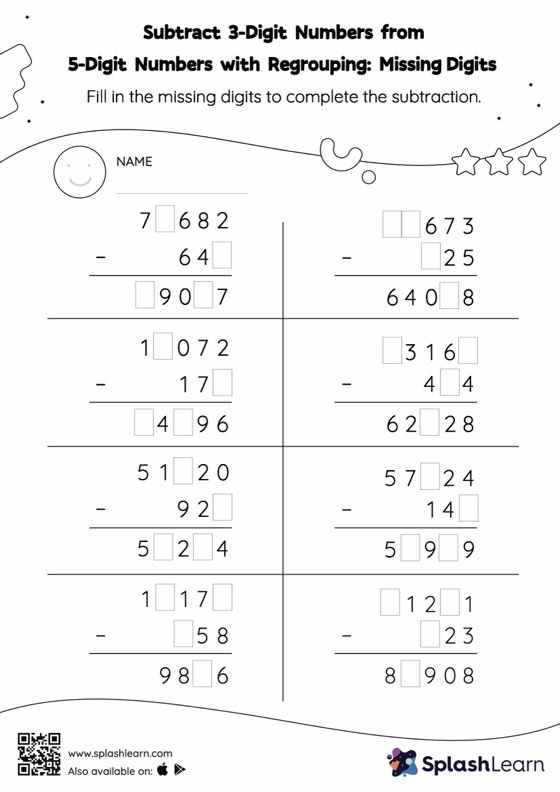# Subtract 3-Digit Numbers from 5-Digit Numbers with Regrouping: Missing Digits Worksheet

Home > Subtract 3-Digit Numbers from 5-Digit Numbers with Regrouping: Missing DigitsTo find the missing number on this worksheet, students can utilize the count back strategy or the connection between addition and subtraction. In subtract 3-digit numbers from 5-digit numbers with regrouping worksheet, they must additionally regroup numbers to reach the solution. As the worksheet uses the column method, it is helpful in getting students toward higher accuracy, especially with bigger numbers and in scenarios where regrouping is required.